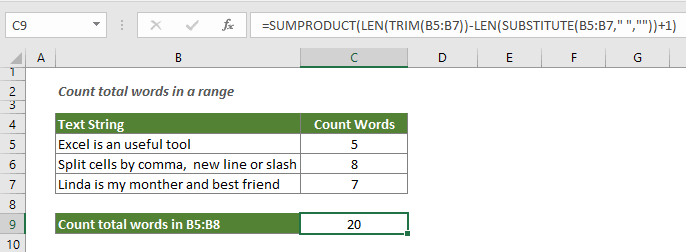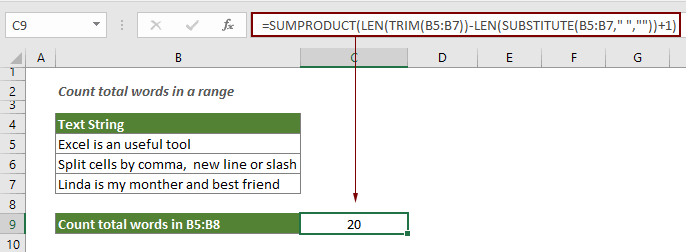## Count total words in a range of cells

This article explains how to count total words in a specific range with a formula in Excel.#### How to count total words in a range of cells in Excel?

Generic formula

=SUMPRODUCT(LEN(TRIM(range))-LEN(SUBSTITUTE(range," ",""))+1)

Argument

Range: The range for which you want to count total words.

How to use this formula?

1. Select a blank cell to output the result.

2. Enter the below formula into it and press the Enter key.

=SUMPRODUCT(LEN(TRIM(B5:B7))-LEN(SUBSTITUTE(B5:B7," ",""))+1)Note: Please change the range B5:B7 in the formula to your need.

How this formula works?

=SUMPRODUCT(LEN(TRIM(B5:B7))-LEN(SUBSTITUTE(B5:B7," ",""))+1)

• 1. LEN(SUBSTITUTE(B5:B7," ","")): The SUBSTITUTE function removes all spaces from the text strings in range B5:B7, and then the LEN function calculates the length of the text strings without spaces and returns the length as an array of numbers: {19;32;29};
• 2. LEN(TRIM(B5:B7)): If there are extra spaces in range B5:B7, the TRIM function will remove them and only keep a single space between words. And then the LEN function calculates the length of the text string in B5:B7 with spaces and return an array of numbers: {23;39;35};
• 3. LEN(TRIM(B5:B7))-LEN(SUBSTITUTE(B5:B7," ","")) = {23;39;35}-{19;32;29}: Now the length of the string with spaces subtracts the string length without spaces and returns the result as an array of numbers: {4;7;6};
• 4. SUMPRODUCT({4;7;6}+1): Here every number in the array is added number 1. That is because the number of words is equal to the number of spaces + 1, we need to add 1 to the final word count. And the SUMPRODUCT function sums the numbers in the array and returns the total words range B5:B7: SUMPRODUCT({5;8;7})=20.

#### Related functions

Excel LEN function
The LEN function returns the number of characters in a text string.

Excel SUBSTITUTE function
The SUBSTITUTE function replaces text or characters within a text string with another text or characters.

Excel SUMPRODUCT function
The SUMPRODUCT function can be used to multiply two or more columns or arrays together, and then get the sum of products.

Excel TRIM function
The Excel TRIM function removes all extra spaces from text string and only keeps single spaces between words.

#### Related formulas

Count occurrences of specific characters in an Excel cell
This tutorial introduces how to apply formulas based on the LEN and SUSTITUTE functions to count the number of times a specific character appears in an Excel cell.

Count specific characters in a range of cells
This tutorial introduces some formulas to count the number of times a specific character appears in a range in Excel.

Count specific words in a cell in Excel

Count specific words in a range in Excel
This article explains a formula to count the number of times a specific word appears in a range of cells in Excel.

Count number of characters in a cell in Excel
The total characters not only includes all letters, but also all spaces, punctuation marks and symbols in the cell. Follow this tutorial to easily count the total characters in a cell with the LEN function in Excel.

Count the number of words in a cell in Excel
This article explains how to count total words in a cell with a formula in Excel.

### The Best Office Productivity Tools

#### Kutools for Excel - Helps You To Stand Out From Crowd

Would you like to complete your daily work quickly and perfectly? Kutools for Excel brings 300 powerful advanced features (Combine workbooks, sum by color, split cell contents, convert date, and so on...) and save 80% time for you.

• Designed for 1500 work scenarios, helps you solve 80% Excel problems.
• Reduce thousands of keyboard and mouse clicks every day, relieve your tired eyes and hands.
• Become an Excel expert in 3 minutes. No longer need to remember any painful formulas and VBA codes.
• 30-day unlimited free trial. 60-day money back guarantee. Free upgrade and support for 2 years.#### Office Tab - Enable Tabbed Reading and Editing in Microsoft Office (include Excel)

• One second to switch between dozens of open documents!
• Reduce hundreds of mouse clicks for you every day, say goodbye to mouse hand.
• Increases your productivity by 50% when viewing and editing multiple documents.
• Brings Efficient Tabs to Office (include Excel), Just Like Chrome, Firefox, And New Internet Explorer.